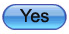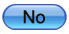Home Math Calculators Math  Flashcards Math Games Math  Homework Math Lessons Math Terms Math Worksheets Math Tools
You Are In Math Online Flashcards

 1. Please Select Your Level: 2. Please Select Flashcard Type: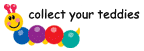Flashcard Type : Addition Level Type : Level 13 + 1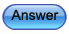Hurray! You have won a loly
3+1=4
You have answered 4; which is correct.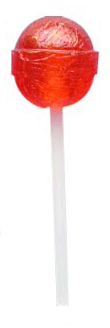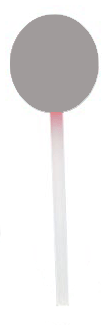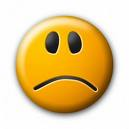Sigh! Your answer is wrong
3+1=4
You have answered 5; which is wrong.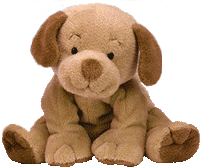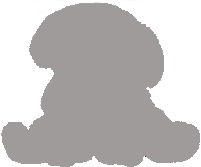Go to Level 2